Normal view MARC view ISBD view

# A comprehensive course in number theory / Alan Baker.

Material type:TextPublisher: New York : Cambridge University Press, 2012Description: xv, 251 p., UKP 22.99 ill. ; 24 cm.ISBN: 9781107019010 (hbk.) :; 110701901X (hbk.) :; 9781107603790 (pbk.) :; 110760379X (pbk.) :.DDC classification: 512.7 Online resources: Cover image
Contents:
Machine generated contents note: Preface; Introduction; 1. Divisibility; 2. Arithmetical functions; 3. Congruences; 4. Quadratic residues; 5. Quadratic forms; 6. Diophantine approximation; 7. Quadratic fields; 8. Diophantine equations; 9. Factorization and primality testing; 10. Number fields; 11. Ideals; 12. Units and ideal classes; 13. Analytic number theory; 14. On the zeros of the zeta-function; 15. On the distribution of the primes; 16. The sieve and circle methods; 17. Elliptic curves; Bibliography; Index.
Summary: "Developed from the author's popular text, A Concise Introduction to the Theory of Numbers, this book provides a comprehensive initiation to all the major branches of number theory. Beginning with the rudiments of the subject, the author proceeds to more advanced topics, including elements of cryptography and primality testing, an account of number fields in the classical vein including properties of their units, ideals and ideal classes, aspects of analytic number theory including studies of the Riemann zeta-function, the prime-number theorem and primes in arithmetical progressions, a description of the Hardy-Littlewood and sieve methods from respectively additive and multiplicative number theory and an exposition of the arithmetic of elliptic curves. The book includes many worked examples, exercises and further reading. Its wider coverage and versatility make this book suitable for courses extending from the elementary to beginning graduate studies"--
Item type Current location Call number Status Date due Barcode Item holds
Book Chennai Mathematical Institute
General Stacks
512.7 BAK (Browse shelf) Available 8546
Total holds: 0
##### Browsing Chennai Mathematical Institute Shelves , Shelving location: General Stacks Close shelf browser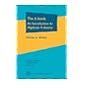No cover image available No cover image available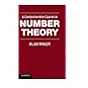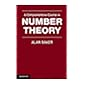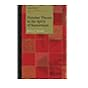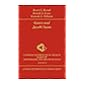512.66 WEG K-theory and C*-algebras : a friendly approach / 512.66 WEI The K-book : an introduction to algebraic K-theory / 512.7 BAA Aperiodic order / 512.7 BAK A comprehensive course in number theory / 512.7 BAK A comprehensive course in number theory / 512.7 BER Number theory in the spirit of Ramanujan / 512.7 BER Gauss and Jacobi sums /

Formerly CIP. Uk

Includes bibliographical references (p. 240-245) and index.

Machine generated contents note: Preface; Introduction; 1. Divisibility; 2. Arithmetical functions; 3. Congruences; 4. Quadratic residues; 5. Quadratic forms; 6. Diophantine approximation; 7. Quadratic fields; 8. Diophantine equations; 9. Factorization and primality testing; 10. Number fields; 11. Ideals; 12. Units and ideal classes; 13. Analytic number theory; 14. On the zeros of the zeta-function; 15. On the distribution of the primes; 16. The sieve and circle methods; 17. Elliptic curves; Bibliography; Index.

"Developed from the author's popular text, A Concise Introduction to the Theory of Numbers, this book provides a comprehensive initiation to all the major branches of number theory. Beginning with the rudiments of the subject, the author proceeds to more advanced topics, including elements of cryptography and primality testing, an account of number fields in the classical vein including properties of their units, ideals and ideal classes, aspects of analytic number theory including studies of the Riemann zeta-function, the prime-number theorem and primes in arithmetical progressions, a description of the Hardy-Littlewood and sieve methods from respectively additive and multiplicative number theory and an exposition of the arithmetic of elliptic curves. The book includes many worked examples, exercises and further reading. Its wider coverage and versatility make this book suitable for courses extending from the elementary to beginning graduate studies"--